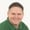Lessons: 11Length: 46 minutes• Overview
• Transcript

# 3.2 Destructuring

We've been able to destructure arrays in PHP for a long time, but 7.1 introduces new syntax for destructuring, as well as the ability to destructure data structures.

## 4.Conclusion1 lesson, 01:09

### 3.2 Destructuring

PHP has had the ability to destructure arrays for a very long time. But with PHP 7.1, we have some new features that allows us to basically do the same thing, but with a better syntax, or at least in my opinion it's a better syntax. But it also changes the behavior of the list construct. Now the list construct has been available since PHP 4 and the idea is pretty simple. We take an array and we specify the variables inside of list that we want to destructure that array. So we can have an array called names. And let's just have three values. So we'll have jeremy, let's have nancy, and then let's have steve. So three names, we want to assign the variables a, b, and c to the values in our names array and that's all that we have to do. So then we can check to see what one of these values are, let's look at A, whenever we run this code, we see that it is Jeremy and if we try to look at the C variable, we see Steve. So this is how we've been able to destructure arrays in PHP. Now with PHP 7, the list construct behaves differently. It starts with the left most parameter and goes to the right. Whereas before hand it started with the right most parameter and went to the left. So there is that difference. But with seven point one we have some new syntax. For destructuring an array, and that's basically using an array like syntax. We have a pair of square brackets. But then we define the variables that we want equal to our array, and we will end up with the same results. Now Visual Studio code did not like that syntax but it works, so never mind that. Now with 7.1, we also have the ability to destructure an array based upon some keys. So let's create another names array. Let's comment out the one that we have and in this case we're going to just have two sets of keys and values. So we're going to say names equals, for our first key let's have first name and we will have that as John. And then we will have a key of last name and we will set that to doe. So we have this nice little data structure, what if we wanted to destructure this into just a couple of variables? Well, We would essentially do the same thing. And we can use either the list function or we can use the new square bracket syntax. So let's look at what that would look like in both cases. As far as list is concerned, we would basically say that okay. We want to take the first name key. And then assign that to the variable first and then we would want to do the same thing with lastName. Except that we're going to assign that to the variable called last. And then we would set to that equal to names and instead of var dumping c let's var dump first. And so we will see in the console that the value of that is John. But let's also just prove that it's not positional. Let's take the last name, and make that where the first name was and vice versa so last name Is going to be called first and so whenever we see that we get the last name. So it's not positional it is going based upon the keys that we have defined. Now we can do this same thing using our new square brackets syntax. So let's just copy and paste that code. We will replace the call to list with our square brackets and we will end up with the same results. Now we can also use this inside of a loop as well. So let's comment out all of this code and let's create an array of people. Each one of these are going to be a data structure similar to what we had with the last name and first name. So, let's just copy that and paste it a few times. Now we have John Doe, let's have Jane Doe. And then let's have something completely different. Let's have Steven Smith. And so these are the values that we want to get inside of our loops so we can have a for each and we're going to use our people as. In here we can use that new destructuring syntax. So that we can say, okay first name is going to be called first, last name is going to be called last. And so we can just write that out very easily with echo and the let's say first and then last and we will separate those with a pair of colons. So whenever we run this, we're going to see John Doe, Jane Doe and then Steven Smith. So when it comes to destructuring arrays in PHP 7.1 it's a lot like what we have been doing except that we have new syntax to make it a little bit easier and more concise. But we also have the added benefit of being able to destructure data structures based upon their keys.

Back to the top